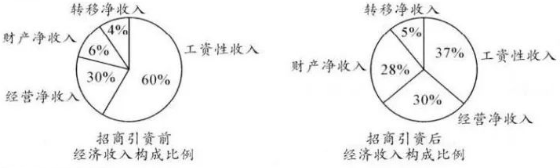$\text{A.}$ 招商引资后,工资性收人较前一年增加 $\text{B.}$ 招商引资后,转移净收人是前一年的 1.25 倍 $\text{C.}$ 招商引资后, 转移净收人与财产净收人的总和超过了该年经济收人的 $\frac{2}{5}$ $\text{D.}$ 招商引资后, 经营净收人较前一年增加了一倍